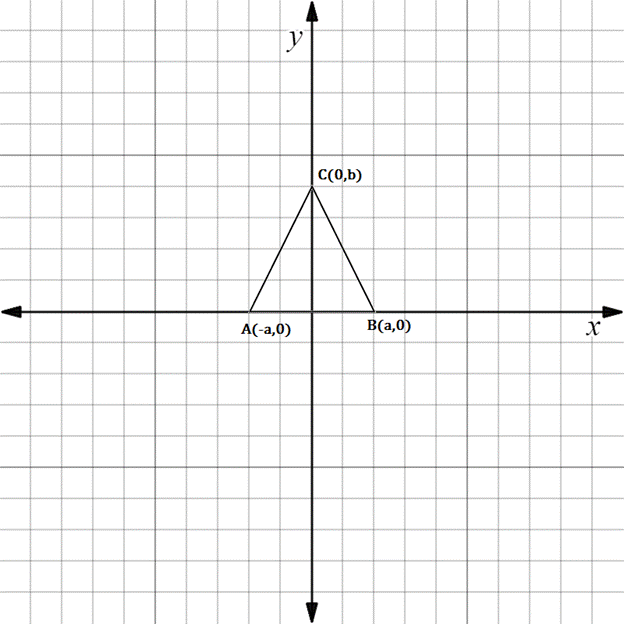Chapter 10.3, Problem 29EElementary Geometry For College St...

7th Edition
Alexander + 2 others
ISBN: 9781337614085

Solutions

Chapter
SectionElementary Geometry For College St...

7th Edition
Alexander + 2 others
ISBN: 9781337614085
Textbook Problem

The drawing shows isosceles △ A B C with A C - ≅ B C - .a) What type of number is a ?b) What type of number is – a ?c) Find an expression for the length of A B - .To determine

(a)

To find:

The type of number a using the given isosceles triangle.

Explanation

The given figure is shown below.

To determine

(b)

To find:

The type of number -a using the given isosceles triangle.

To determine

(c)

To find:

The expression for the length AB-.

Still sussing out bartleby?

Check out a sample textbook solution.

See a sample solution

The Solution to Your Study Problems

Bartleby provides explanations to thousands of textbook problems written by our experts, many with advanced degrees!

Get Started

Differentiate. y = 2 sec x csc x

Calculus: Early Transcendentals

Find the derivative. Simplify where possible. 40. y = sinh1(tan x)

Single Variable Calculus: Early Transcendentals, Volume I

Simplify the expressions in Exercises 97106. x3/2x5/2

Finite Mathematics and Applied Calculus (MindTap Course List)

Find the derivative of the function. f(t)=sin2(esin2t)

Single Variable Calculus: Early Transcendentals